Dimitri Lozeve's Blog https://www.lozeve.com/atom.xml Dimitri Lozeve dimitri+web@lozeve.com 2019-04-06T00:00:00Z Mindsay: Towards Self-Learning Chatbots https://www.lozeve.com/posts/self-learning-chatbots-destygo.html 2019-04-06T00:00:00Z 2019-04-06T00:00:00Z
Posted on April 6, 2019

Last week I made a presentation at the Paris NLP Meetup, on how we implemented self-learning chatbots in production at Mindsay.

It was fascinating to other people interested in NLP about the technologies and models we deploy at work! It’s always nice to have some feedback about our work, and preparing this talk forced me to take a step back about what we do and rethink it in new interesting ways.

Also check out the other presentations, one about diachronic (i.e. time-dependent) word embeddings and the other about the different models and the use of Knowledge Bases for Information Retrieval. (This might even give us new ideas to explore…)

If you’re interested about exciting applications at the confluence of Reinforcement Learning and NLP, the slides are available here. It includes basic RL theory, how we transposed it to the specific case of conversational agents, the technical and mathematical challenges in implementing self-learning chatbots, and of course plenty of references for further reading if we piqued your interest!

Update: The videos are now available on the NLP Meetup website.

Update 2: Destygo changed its name to Mindsay!

]]>
Random matrices from the Ginibre ensemble https://www.lozeve.com/posts/ginibre-ensemble.html 2019-03-20T00:00:00Z 2019-03-20T00:00:00Z
Posted on March 20, 2019

## Ginibre ensemble and its properties

The Ginibre ensemble is a set of random matrices with the entries chosen independently. Each entry of a $$n \times n$$ matrix is a complex number, with both the real and imaginary part sampled from a normal distribution of mean zero and variance $$1/2n$$.

Random matrices distributions are very complex and are a very active subject of research. I stumbled on this example while reading an article in Notices of the AMS by Brian C. Hall (1).

Now what is interesting about these random matrices is the distribution of their $$n$$ eigenvalues in the complex plane.

The circular law (first established by Jean Ginibre in 1965 (2)) states that when $$n$$ is large, with high probability, almost all the eigenvalues lie in the unit disk. Moreover, they tend to be nearly uniformly distributed there.

I find this mildly fascinating that such a straightforward definition of a random matrix can exhibit such non-random properties in their spectrum.

## Simulation

I ran a quick simulation, thanks to Julia’s great ecosystem for linear algebra and statistical distributions:

using LinearAlgebra
using UnicodePlots

function ginibre(n)
return randn((n, n)) * sqrt(1/2n) + im * randn((n, n)) * sqrt(1/2n)
end

v = eigvals(ginibre(2000))

scatterplot(real(v), imag(v), xlim=[-1.5,1.5], ylim=[-1.5,1.5])

I like using UnicodePlots for this kind of quick-and-dirty plots, directly in the terminal. Here is the output: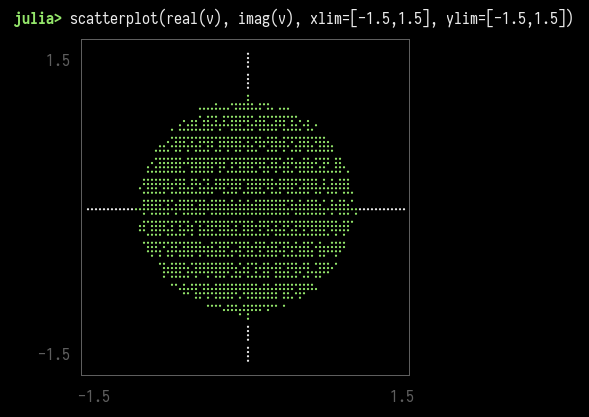1. Hall, Brian C. 2019. “Eigenvalues of Random Matrices in the General Linear Group in the Large-$$N$$ Limit.” Notices of the American Mathematical Society 66, no. 4 (Spring): 568-569. https://www.ams.org/journals/notices/201904/201904FullIssue.pdf
2. Ginibre, Jean. “Statistical ensembles of complex, quaternion, and real matrices.” Journal of Mathematical Physics 6.3 (1965): 440-449. https://doi.org/10.1063/1.1704292
Peano Axioms https://www.lozeve.com/posts/peano.html 2019-03-18T00:00:00Z 2019-03-18T00:00:00Z
Posted on March 18, 2019

# Introduction

I have recently bought the book Category Theory from Steve Awodey (Awodey 2010) is awesome, but probably the topic for another post), and a particular passage excited my curiosity:

Let us begin by distinguishing between the following things: i. categorical foundations for mathematics, ii. mathematical foundations for category theory.

As for the first point, one sometimes hears it said that category theory can be used to provide “foundations for mathematics,” as an alternative to set theory. That is in fact the case, but it is not what we are doing here. In set theory, one often begins with existential axioms such as “there is an infinite set” and derives further sets by axioms like “every set has a powerset,” thus building up a universe of mathematical objects (namely sets), which in principle suffice for “all of mathematics.”

This statement is interesting because one often considers category theory as pretty “fundamental”, in the sense that it has no issue with considering what I call “dangerous” notions, such as the category $$\mathbf{Set}$$ of all sets, and even the category $$\mathbf{Cat}$$ of all categories. Surely a theory this general, that can afford to study such objects, should provide suitable foundations for mathematics? Awodey addresses these issues very explicitly in the section following the quote above, and finds a good way of avoiding circular definitions.

Now, I remember some basics from my undergrad studies about foundations of mathematics. I was told that if you could define arithmetic, you basically had everything else “for free” (as Kronecker famously said, “natural numbers were created by God, everything else is the work of men”). I was also told that two sets of axioms existed, the Peano axioms and the Zermelo-Fraenkel axioms. Also, I should steer clear of the axiom of choice if I could, because one can do strange things with it, and it is equivalent to many different statements. Finally (and this I knew mainly from Logicomix, I must admit), it is impossible for a set of axioms to be both complete and consistent.

Given all this, I realised that my knowledge of foundational mathematics was pretty deficient. I do not believe that it is a very important topic that everyone should know about, even though Gödel’s incompleteness theorem is very interesting from a logical and philosophical standpoint. However, I wanted to go deeper on this subject.

In this post, I will try to share my path through Peano’s axioms (Gowers, Barrow-Green, and Leader 2010), because they are very simple, and it is easy to uncover basic algebraic structure from them.

# The Axioms

The purpose of the axioms is to define a collection of objects that we will call the natural numbers. Here, we place ourselves in the context of first-order logic. Logic is not the main topic here, so I will just assume that I have access to some quantifiers, to some predicates, to some variables, and, most importantly, to a relation $$=$$ which is reflexive, symmetric, transitive, and closed over the natural numbers.

Without further digressions, let us define two symbols $$0$$ and $$s$$ (called successor) such that:

1. $$0$$ is a natural number.
2. For every natural number $$n$$, $$s(n)$$ is a natural number. (“The successor of a natural number is a natural number.”)
3. For all natural number $$m$$ and $$n$$, if $$s(m) = s(n)$$, then $$m=n$$. (“If two numbers have the same successor, they are equal.”)
4. For every natural number $$n$$, $$s(n) = 0$$ is false. (“$$0$$ is nobody’s successor.”)
5. If $$A$$ is a set such that:
• $$0$$ is in $$A$$
• for every natural number $$n$$, if $$n$$ is in $$A$$ then $$s(n)$$ is in $$A$$
then $$A$$ contains every natural number.

Let’s break this down. Axioms 1–4 define a collection of objects, written $$0$$, $$s(0)$$, $$s(s(0))$$, and so on, and ensure their basic properties. All of these are natural numbers by the first four axioms, but how can we be sure that all natural numbers are of the form $$s( \cdots s(0))$$? This is where the induction axiom (Axiom 5) intervenes. It ensures that every natural number is “well-formed” according to the previous axioms.

But Axiom 5 is slightly disturbing, because it mentions a “set” and a relation “is in”. This seems pretty straightforward at first sight, but these notions were never defined anywhere before that! Isn’t our goal to define all these notions in order to derive a foundation of mathematics? (I still don’t know the answer to that question.) I prefer the following alternative version of the induction axiom:

• If $$\varphi$$ is a unary predicate such that:
• $$\varphi(0)$$ is true
• for every natural number $$n$$, if $$\varphi(n)$$ is true, then $$\varphi(s(n))$$ is also true
then $$\varphi(n)$$ is true for every natural number $$n$$.

The alternative formulation is much better in my opinion, as it obviously implies the first one (juste choose $$\varphi(n)$$ as “$$n$$ is a natural number”), and it only references predicates. It will also be much more useful afterwards, as we will see.

What is needed afterwards? The most basic notion after the natural numbers themselves is the addition operator. We define an operator $$+$$ by the following (recursive) rules:

1. $$\forall a,\quad a+0 = a$$.
2. $$\forall a, \forall b,\quad a + s(b) = s(a+b)$$.

Let us use these rules to prove the basic properties of $$+$$.

## Commutativity

$$\forall a, \forall b,\quad a+b = b+a$$.

First, we prove that every natural number commutes with $$0$$.

• $$0+0 = 0+0$$.
• For every natural number $$a$$ such that $$0+a = a+0$$, we have:

\begin{align} 0 + s(a) &= s(0+a)\\ &= s(a+0)\\ &= s(a)\\ &= s(a) + 0. \end{align}

By Axiom 5, every natural number commutes with $$0$$.

We can now prove the main proposition:

• $$\forall a,\quad a+0=0+a$$.
• For all $$a$$ and $$b$$ such that $$a+b=b+a$$,

\begin{align} a + s(b) &= s(a+b)\\ &= s(b+a)\\ &= s(b) + a. \end{align}

We used the opposite of the second rule for $$+$$, namely $$\forall a, \forall b,\quad s(a) + b = s(a+b)$$. This can easily be proved by another induction.

## Associativity

$$\forall a, \forall b, \forall c,\quad a+(b+c) = (a+b)+c$$.

Todo, left as an exercise to the reader 😉

## Identity element

$$\forall a,\quad a+0 = 0+a = a$$.

This follows directly from the definition of $$+$$ and commutativity.

From all these properties, it follows that the set of natural numbers with $$+$$ is a commutative monoid.

# Going further

We have imbued our newly created set of natural numbers with a significant algebraic structure. From there, similar arguments will create more structure, notably by introducing another operation $$\times$$, and an order $$\leq$$.

It is now a matter of conventional mathematics to construct the integers $$\mathbb{Z}$$ and the rationals $$\mathbb{Q}$$ (using equivalence classes), and eventually the real numbers $$\mathbb{R}$$.

It is remarkable how very few (and very simple, as far as you would consider the induction axiom “simple”) axioms are enough to build an entire theory of mathematics. This sort of things makes me agree with Eugene Wigner (Wigner 1990) when he says that “mathematics is the science of skillful operations with concepts and rules invented just for this purpose”. We drew some arbitrary rules out of thin air, and derived countless properties and theorems from them, basically for our own enjoyment. (As Wigner would say, it is incredible that any of these fanciful inventions coming out of nowhere turned out to be even remotely useful.) Mathematics is done mainly for the mathematician’s own pleasure!

Mathematics cannot be defined without acknowledging its most obvious feature: namely, that it is interesting — M. Polanyi (Wigner 1990)

# References

Awodey, Steve. 2010. Category Theory. 2nd ed. Oxford Logic Guides 52. Oxford ; New York: Oxford University Press.

Gowers, Timothy, June Barrow-Green, and Imre Leader. 2010. The Princeton Companion to Mathematics. Princeton University Press.

Wigner, Eugene P. 1990. “The Unreasonable Effectiveness of Mathematics in the Natural Sciences.” In Mathematics and Science, by Ronald E Mickens, 291–306. WORLD SCIENTIFIC. https://doi.org/10.1142/9789814503488_0018.

]]>
Quick Notes on Reinforcement Learning https://www.lozeve.com/posts/reinforcement-learning-1.html 2018-11-21T00:00:00Z 2018-11-21T00:00:00Z
Posted on November 21, 2018

# Introduction

In this series of blog posts, I intend to write my notes as I go through Richard S. Sutton’s excellent Reinforcement Learning: An Introduction (1).

I will try to formalise the maths behind it a little bit, mainly because I would like to use it as a useful personal reference to the main concepts in RL. I will probably add a few remarks about a possible implementation as I go on.

# Relationship between agent and environment

## Context and assumptions

The goal of reinforcement learning is to select the best actions availables to an agent as it goes through a series of states in an environment. In this post, we will only consider discrete time steps.

The most important hypothesis we make is the Markov property:

At each time step, the next state of the agent depends only on the current state and the current action taken. It cannot depend on the history of the states visited by the agent.

This property is essential to make our problems tractable, and often holds true in practice (to a reasonable approximation).

With this assumption, we can define the relationship between agent and environment as a Markov Decision Process (MDP).

A Markov Decision Process is a tuple $$(\mathcal{S}, \mathcal{A}, \mathcal{R}, p)$$ where:

• $$\mathcal{S}$$ is a set of states,
• $$\mathcal{A}$$ is an application mapping each state $$s \in \mathcal{S}$$ to a set $$\mathcal{A}(s)$$ of possible actions for this state. In this post, we will often simplify by using $$\mathcal{A}$$ as a set, assuming that all actions are possible for each state,
• $$\mathcal{R} \subset \mathbb{R}$$ is a set of rewards,
• and $$p$$ is a function representing the dynamics of the MDP:

\begin{align} p &: \mathcal{S} \times \mathcal{R} \times \mathcal{S} \times \mathcal{A} \mapsto [0,1] \\ p(s', r \;|\; s, a) &:= \mathbb{P}(S_t=s', R_t=r \;|\; S_{t-1}=s, A_{t-1}=a), \end{align}

such that $\forall s \in \mathcal{S}, \forall a \in \mathcal{A},\quad \sum_{s', r} p(s', r \;|\; s, a) = 1.$

The function $$p$$ represents the probability of transitioning to the state $$s'$$ and getting a reward $$r$$ when the agent is at state $$s$$ and chooses action $$a$$.

We will also use occasionally the state-transition probabilities:

\begin{align} p &: \mathcal{S} \times \mathcal{S} \times \mathcal{A} \mapsto [0,1] \\ p(s' \;|\; s, a) &:= \mathbb{P}(S_t=s' \;|\; S_{t-1}=s, A_{t-1}=a) \\ &= \sum_r p(s', r \;|\; s, a). \end{align}

## Rewarding the agent

The expected reward of a state-action pair is the function

\begin{align} r &: \mathcal{S} \times \mathcal{A} \mapsto \mathbb{R} \\ r(s,a) &:= \mathbb{E}[R_t \;|\; S_{t-1}=s, A_{t-1}=a] \\ &= \sum_r r \sum_{s'} p(s', r \;|\; s, a). \end{align}

The discounted return is the sum of all future rewards, with a multiplicative factor to give more weights to more immediate rewards: $G_t := \sum_{k=t+1}^T \gamma^{k-t-1} R_k,$ where $$T$$ can be infinite or $$\gamma$$ can be 1, but not both.

# Deciding what to do: policies

## Defining our policy and its value

A policy is a way for the agent to choose the next action to perform.

A policy is a function $$\pi$$ defined as

\begin{align} \pi &: \mathcal{A} \times \mathcal{S} \mapsto [0,1] \\ \pi(a \;|\; s) &:= \mathbb{P}(A_t=a \;|\; S_t=s). \end{align}

In order to compare policies, we need to associate values to them.

The state-value function of a policy $$\pi$$ is

\begin{align} v_{\pi} &: \mathcal{S} \mapsto \mathbb{R} \\ v_{\pi}(s) &:= \text{expected return when starting in s and following \pi} \\ v_{\pi}(s) &:= \mathbb{E}_{\pi}\left[ G_t \;|\; S_t=s\right] \\ v_{\pi}(s) &= \mathbb{E}_{\pi}\left[ \sum_{k=0}^{\infty} \gamma^k R_{t+k+1} \;|\; S_t=s\right] \end{align}

We can also compute the value starting from a state $$s$$ by also taking into account the action taken $$a$$.

The action-value function of a policy $$\pi$$ is

\begin{align} q_{\pi} &: \mathcal{S} \times \mathcal{A} \mapsto \mathbb{R} \\ q_{\pi}(s,a) &:= \text{expected return when starting from s, taking action a, and following \pi} \\ q_{\pi}(s,a) &:= \mathbb{E}_{\pi}\left[ G_t \;|\; S_t=s, A_t=a \right] \\ q_{\pi}(s,a) &= \mathbb{E}_{\pi}\left[ \sum_{k=0}^{\infty} \gamma^k R_{t+k+1} \;|\; S_t=s, A_t=a\right] \end{align}

# References

1. R. S. Sutton and A. G. Barto, Reinforcement learning: an introduction, Second edition. Cambridge, MA: The MIT Press, 2018.
]]>
Ising model simulation in APL https://www.lozeve.com/posts/ising-apl.html 2018-03-05T00:00:00Z 2018-03-05T00:00:00Z
Posted on March 5, 2018

# The APL family of languages

## Why APL?

I recently got interested in APL, an array-based programming language. In APL (and derivatives), we try to reason about programs as series of transformations of multi-dimensional arrays. This is exactly the kind of style I like in Haskell and other functional languages, where I also try to use higher-order functions (map, fold, etc) on lists or arrays. A developer only needs to understand these abstractions once, instead of deconstructing each loop or each recursive function encountered in a program.

APL also tries to be a really simple and terse language. This combined with strange Unicode characters for primitive functions and operators, gives it a reputation of unreadability. However, there is only a small number of functions to learn, and you get used really quickly to read them and understand what they do. Some combinations also occur so frequently that you can recognize them instantly (APL programmers call them idioms).

## Implementations

APL is actually a family of languages. The classic APL, as created by Ken Iverson, with strange symbols, has many implementations. I initially tried GNU APL, but due to the lack of documentation and proper tooling, I went to Dyalog APL (which is proprietary, but free for personal use). There are also APL derivatives, that often use ASCII symbols: J (free) and Q/kdb+ (proprietary, but free for personal use).

The advantage of Dyalog is that it comes with good tooling (which is necessary for inserting all the symbols!), a large ecosystem, and pretty good documentation. If you want to start, look at Mastering Dyalog APL by Bernard Legrand, freely available online.

# The Ising model in APL

I needed a small project to try APL while I was learning. Something array-based, obviously. Since I already implemented a Metropolis-Hastings simulation of the Ising model, which is based on a regular lattice, I decided to reimplement it in Dyalog APL.

It is only a few lines long, but I will try to explain what it does step by step.

The first function simply generates a random lattice filled by elements of $$\{-1,+1\}$$.

L←{(2×?⍵ ⍵⍴2)-3}


Let’s deconstruct what is done here:

• ⍵ is the argument of our function.
• We generate a ⍵×⍵ matrix filled with 2, using the ⍴ function: ⍵ ⍵⍴2
• ? draws a random number between 1 and its argument. We give it our matrix to generate a random matrix of 1 and 2.
• We multiply everything by 2 and subtract 3, so that the result is in $$\{-1,+1\}$$.
• Finally, we assign the result to the name L.

Sample output:

      ising.L 5
1 ¯1  1 ¯1  1
1  1  1 ¯1 ¯1
1 ¯1 ¯1 ¯1 ¯1
1  1  1 ¯1 ¯1
¯1 ¯1  1  1  1


Next, we compute the energy variation (for details on the Ising model, see my previous post).

∆E←{
⎕IO←0
(x y)←⍺
N←⊃⍴⍵
xn←N|((x-1)y)((x+1)y)
yn←N|(x(y-1))(x(y+1))
⍵[x;y]×+/⍵[xn,yn]
}

• ⍺ is the left argument (coordinates of the site), ⍵ is the right argument (lattice).
• We extract the x and y coordinates of the site.
• N is the size of the lattice.
• xn and yn are respectively the vertical and lateral neighbours of the site. N| takes the coordinates modulo N (so the lattice is actually a torus). (Note: we used ⎕IO←0 to use 0-based array indexing.)
• +/ sums over all neighbours of the site, and then we multiply by the value of the site itself to get $$\Delta E$$.

Sample output, for site $$(3, 3)$$ in a random $$5\times 5$$ lattice:

      3 3ising.∆E ising.L 5
¯4


Then comes the actual Metropolis-Hastings part:

U←{
⎕IO←0
N←⊃⍴⍵
(x y)←?N N
new←⍵
new[x;y]×←(2×(?0)>*-⍺×x y ∆E ⍵)-1
new
}

• ⍺ is the $$\beta$$ parameter of the Ising model, ⍵ is the lattice.
• We draw a random site $$(x,y)$$ with the ? function.
• new is the lattice but with the $$(x,y)$$ site flipped.
• We compute the probability $$\alpha = \exp(-\beta\Delta E)$$ using the * function (exponential) and our previous ∆E function.
• ?0 returns a uniform random number in $$[0,1)$$. Based on this value, we decide whether to update the lattice, and we return it.

We can now bring everything together for display:

Ising←{' ⌹'[1+1=({10 U ⍵}⍣⍵)L ⍺]}

• We draw a random lattice of size ⍺ with L ⍺.
• We apply to it our update function, with $β$=10, ⍵ times (using the ⍣ function, which applies a function $$n$$ times.
• Finally, we display -1 as a space and 1 as a domino ⌹.

Final output, with a $$80\times 80$$ random lattice, after 50000 update steps:

      80ising.Ising 50000
⌹⌹⌹⌹ ⌹⌹⌹⌹⌹⌹⌹⌹⌹⌹      ⌹⌹⌹⌹⌹⌹       ⌹⌹⌹⌹⌹      ⌹⌹⌹⌹       ⌹⌹⌹⌹⌹⌹⌹⌹⌹⌹⌹
⌹⌹⌹⌹      ⌹⌹⌹⌹⌹⌹     ⌹⌹⌹⌹⌹        ⌹⌹⌹⌹⌹      ⌹⌹⌹⌹⌹⌹ ⌹⌹⌹⌹⌹⌹⌹⌹⌹⌹⌹⌹⌹⌹
⌹⌹⌹⌹⌹⌹⌹      ⌹⌹⌹⌹⌹⌹       ⌹⌹⌹     ⌹⌹⌹⌹⌹⌹⌹⌹       ⌹⌹⌹⌹⌹⌹⌹⌹⌹⌹⌹⌹⌹⌹⌹⌹⌹⌹            ⌹
⌹⌹⌹⌹⌹⌹⌹      ⌹⌹⌹⌹⌹⌹       ⌹⌹⌹  ⌹⌹⌹⌹⌹⌹⌹⌹⌹⌹⌹⌹         ⌹⌹⌹⌹⌹⌹⌹⌹⌹⌹⌹⌹⌹⌹⌹            ⌹
⌹⌹⌹⌹⌹⌹⌹⌹⌹      ⌹⌹⌹⌹            ⌹⌹⌹⌹⌹⌹⌹⌹⌹⌹⌹⌹⌹⌹⌹⌹     ⌹⌹⌹⌹⌹⌹⌹⌹⌹⌹⌹⌹⌹⌹             ⌹
⌹⌹⌹⌹⌹⌹⌹⌹⌹      ⌹⌹⌹⌹            ⌹⌹⌹⌹⌹⌹⌹⌹⌹⌹⌹⌹⌹⌹⌹⌹⌹⌹   ⌹⌹⌹     ⌹⌹⌹⌹⌹⌹             ⌹
⌹⌹⌹⌹⌹⌹⌹⌹⌹⌹⌹⌹⌹⌹ ⌹⌹⌹            ⌹⌹⌹⌹⌹⌹⌹⌹⌹⌹⌹⌹⌹⌹⌹⌹⌹⌹⌹   ⌹⌹       ⌹⌹⌹⌹⌹      ⌹
⌹⌹⌹⌹⌹⌹⌹⌹⌹⌹⌹⌹⌹⌹⌹⌹⌹⌹          ⌹⌹⌹⌹⌹⌹⌹⌹⌹⌹⌹⌹⌹⌹⌹⌹⌹⌹⌹   ⌹        ⌹⌹⌹⌹      ⌹⌹⌹
⌹⌹⌹⌹⌹⌹⌹⌹⌹⌹⌹⌹⌹⌹⌹⌹⌹⌹⌹⌹⌹⌹⌹      ⌹⌹⌹⌹⌹⌹⌹⌹⌹⌹  ⌹⌹⌹⌹⌹⌹⌹            ⌹⌹⌹       ⌹⌹⌹
⌹⌹⌹⌹⌹⌹⌹⌹⌹⌹⌹⌹⌹⌹⌹⌹⌹⌹⌹⌹⌹⌹⌹      ⌹⌹⌹⌹⌹⌹⌹⌹⌹⌹  ⌹⌹⌹⌹⌹⌹⌹⌹⌹⌹         ⌹⌹⌹    ⌹⌹⌹⌹⌹⌹
⌹⌹⌹⌹⌹⌹⌹⌹⌹⌹⌹⌹⌹⌹⌹⌹⌹⌹⌹⌹⌹⌹⌹⌹      ⌹⌹⌹⌹⌹⌹⌹⌹⌹⌹⌹        ⌹⌹⌹⌹              ⌹⌹⌹⌹⌹⌹⌹⌹⌹⌹⌹⌹⌹
⌹⌹⌹⌹        ⌹⌹⌹⌹⌹⌹⌹⌹⌹⌹⌹⌹      ⌹⌹⌹⌹⌹⌹⌹⌹⌹⌹⌹         ⌹⌹⌹⌹⌹⌹⌹⌹⌹⌹       ⌹⌹⌹⌹⌹⌹⌹⌹⌹⌹⌹⌹⌹
⌹⌹⌹⌹           ⌹⌹⌹⌹⌹⌹⌹⌹⌹⌹⌹    ⌹⌹⌹⌹⌹⌹⌹⌹⌹⌹⌹         ⌹⌹⌹⌹⌹⌹⌹⌹⌹⌹       ⌹⌹⌹⌹⌹⌹⌹⌹⌹⌹⌹⌹⌹
⌹⌹⌹⌹           ⌹⌹⌹⌹⌹⌹⌹⌹⌹⌹⌹            ⌹⌹⌹          ⌹⌹⌹⌹⌹⌹⌹⌹⌹       ⌹⌹⌹⌹⌹⌹⌹⌹⌹⌹⌹⌹⌹
⌹⌹⌹            ⌹⌹⌹⌹⌹⌹⌹⌹⌹⌹⌹            ⌹⌹⌹           ⌹⌹⌹⌹⌹⌹⌹⌹      ⌹⌹⌹⌹⌹⌹⌹⌹⌹⌹⌹⌹⌹⌹
⌹⌹⌹⌹           ⌹⌹⌹⌹⌹⌹⌹⌹⌹⌹⌹            ⌹⌹⌹⌹           ⌹⌹⌹⌹⌹⌹⌹      ⌹⌹⌹⌹⌹⌹⌹  ⌹⌹⌹⌹⌹
⌹⌹⌹⌹ ⌹⌹⌹⌹⌹     ⌹⌹⌹⌹⌹⌹⌹⌹⌹⌹⌹            ⌹⌹⌹            ⌹⌹⌹⌹⌹⌹⌹     ⌹⌹⌹⌹⌹⌹⌹⌹  ⌹⌹⌹⌹⌹
⌹⌹⌹⌹⌹⌹⌹⌹⌹⌹⌹   ⌹⌹⌹⌹⌹⌹⌹⌹⌹⌹⌹⌹           ⌹⌹⌹⌹                ⌹⌹⌹     ⌹⌹⌹⌹⌹⌹⌹    ⌹⌹⌹⌹
⌹ ⌹⌹⌹⌹⌹⌹⌹⌹⌹⌹⌹⌹⌹⌹⌹⌹⌹ ⌹⌹⌹⌹           ⌹⌹⌹⌹                ⌹⌹⌹      ⌹⌹⌹⌹⌹
⌹⌹⌹⌹⌹⌹⌹⌹⌹⌹⌹⌹⌹⌹⌹⌹    ⌹⌹⌹⌹⌹⌹       ⌹⌹⌹⌹⌹⌹⌹                ⌹⌹        ⌹
⌹⌹⌹⌹⌹⌹⌹⌹⌹⌹⌹⌹⌹⌹⌹⌹    ⌹⌹⌹⌹⌹⌹⌹      ⌹⌹⌹⌹⌹⌹⌹⌹
⌹⌹⌹⌹⌹⌹⌹⌹⌹⌹⌹⌹⌹⌹⌹⌹    ⌹⌹⌹⌹⌹⌹⌹⌹     ⌹⌹⌹⌹⌹⌹⌹⌹⌹
⌹⌹⌹⌹⌹⌹⌹⌹⌹⌹⌹⌹⌹⌹⌹⌹⌹⌹  ⌹⌹⌹⌹⌹⌹       ⌹⌹⌹⌹⌹⌹⌹⌹           ⌹
⌹⌹⌹⌹⌹⌹⌹⌹⌹⌹⌹⌹⌹⌹⌹⌹⌹⌹⌹  ⌹⌹⌹⌹⌹⌹        ⌹⌹⌹⌹⌹⌹⌹          ⌹
⌹⌹⌹⌹⌹⌹  ⌹⌹⌹⌹⌹⌹⌹⌹⌹⌹⌹⌹     ⌹⌹⌹          ⌹⌹⌹⌹⌹⌹                          ⌹⌹     ⌹⌹⌹
⌹⌹⌹⌹⌹⌹  ⌹⌹⌹⌹⌹⌹⌹⌹⌹⌹⌹⌹                  ⌹⌹⌹⌹⌹⌹         ⌹               ⌹⌹⌹     ⌹⌹⌹
⌹⌹⌹⌹⌹⌹  ⌹⌹⌹⌹⌹⌹⌹⌹⌹⌹⌹⌹                   ⌹⌹⌹⌹⌹⌹                     ⌹⌹⌹⌹⌹⌹     ⌹⌹⌹
⌹⌹⌹⌹⌹⌹  ⌹⌹⌹⌹⌹⌹⌹⌹⌹⌹⌹⌹       ⌹⌹⌹⌹⌹       ⌹⌹⌹⌹⌹⌹⌹⌹⌹⌹⌹⌹⌹⌹⌹            ⌹⌹⌹⌹⌹⌹    ⌹⌹⌹⌹
⌹⌹⌹⌹⌹⌹⌹⌹⌹⌹⌹⌹⌹⌹⌹⌹⌹⌹⌹⌹     ⌹⌹⌹⌹⌹⌹⌹⌹      ⌹⌹⌹⌹⌹⌹⌹⌹⌹⌹⌹⌹⌹⌹⌹            ⌹⌹⌹⌹⌹⌹⌹⌹⌹⌹⌹⌹⌹⌹
⌹⌹⌹⌹⌹⌹⌹⌹⌹⌹⌹⌹⌹⌹⌹⌹⌹⌹    ⌹⌹⌹⌹⌹⌹⌹⌹⌹⌹⌹       ⌹⌹⌹⌹⌹⌹⌹⌹⌹⌹⌹⌹⌹             ⌹⌹⌹⌹⌹⌹⌹⌹⌹⌹⌹⌹
⌹⌹⌹⌹⌹⌹⌹⌹⌹       ⌹⌹⌹⌹    ⌹⌹⌹⌹⌹⌹⌹⌹⌹⌹⌹⌹⌹     ⌹⌹⌹⌹⌹⌹⌹⌹⌹⌹⌹⌹⌹                ⌹⌹⌹⌹⌹⌹⌹⌹⌹
⌹⌹⌹⌹⌹⌹⌹⌹⌹       ⌹⌹⌹⌹    ⌹⌹⌹⌹⌹⌹⌹⌹⌹⌹⌹⌹⌹    ⌹⌹⌹⌹⌹⌹⌹⌹⌹⌹⌹⌹⌹⌹                 ⌹⌹⌹⌹⌹⌹⌹⌹
⌹⌹⌹⌹⌹⌹⌹⌹⌹       ⌹              ⌹⌹⌹⌹⌹⌹⌹⌹⌹⌹⌹⌹⌹⌹ ⌹⌹⌹⌹⌹⌹⌹⌹⌹              ⌹⌹⌹⌹⌹⌹⌹⌹⌹⌹⌹
⌹⌹⌹⌹⌹⌹⌹⌹⌹                            ⌹⌹⌹⌹⌹⌹⌹    ⌹⌹⌹⌹⌹⌹⌹⌹⌹          ⌹⌹⌹⌹⌹⌹⌹⌹⌹⌹⌹⌹⌹
⌹⌹⌹⌹⌹⌹⌹⌹⌹                               ⌹⌹⌹⌹      ⌹⌹⌹⌹⌹⌹⌹⌹⌹⌹⌹⌹⌹  ⌹⌹⌹⌹⌹⌹⌹⌹⌹⌹⌹⌹⌹⌹⌹
⌹⌹⌹⌹⌹⌹⌹⌹⌹                                ⌹⌹⌹        ⌹⌹⌹⌹⌹⌹⌹⌹⌹⌹⌹    ⌹⌹⌹⌹⌹⌹⌹⌹⌹⌹⌹⌹⌹
⌹⌹⌹⌹⌹⌹                   ⌹⌹⌹⌹             ⌹⌹        ⌹⌹⌹⌹⌹⌹⌹⌹⌹⌹⌹⌹   ⌹⌹⌹⌹⌹⌹⌹⌹⌹⌹⌹⌹⌹
⌹⌹⌹⌹⌹⌹                   ⌹⌹⌹⌹                           ⌹⌹⌹⌹⌹⌹⌹⌹⌹⌹⌹⌹⌹⌹⌹⌹⌹⌹⌹⌹⌹⌹⌹⌹
⌹⌹⌹⌹⌹⌹                  ⌹⌹⌹⌹⌹⌹⌹                ⌹⌹            ⌹⌹⌹⌹⌹⌹⌹⌹⌹⌹⌹⌹⌹⌹⌹⌹⌹⌹
⌹⌹⌹⌹                  ⌹⌹⌹⌹⌹⌹⌹              ⌹⌹⌹⌹              ⌹⌹⌹⌹⌹⌹⌹⌹⌹⌹⌹⌹⌹⌹⌹
⌹⌹⌹⌹                 ⌹⌹⌹⌹⌹⌹⌹⌹⌹             ⌹⌹⌹⌹              ⌹⌹⌹⌹⌹⌹⌹⌹⌹⌹⌹⌹⌹⌹⌹
⌹⌹⌹⌹   ⌹         ⌹⌹⌹⌹⌹⌹⌹⌹⌹⌹⌹⌹⌹⌹⌹         ⌹⌹⌹⌹⌹⌹              ⌹⌹⌹⌹⌹⌹⌹⌹⌹⌹⌹⌹⌹⌹⌹
⌹⌹⌹⌹⌹   ⌹ ⌹       ⌹⌹⌹⌹⌹⌹⌹⌹⌹⌹⌹⌹⌹⌹⌹⌹⌹      ⌹⌹⌹⌹⌹⌹⌹⌹           ⌹⌹⌹⌹⌹⌹⌹⌹⌹⌹⌹⌹⌹⌹⌹⌹⌹
⌹⌹⌹⌹⌹   ⌹         ⌹⌹⌹⌹⌹⌹⌹⌹⌹⌹⌹⌹⌹⌹⌹⌹⌹      ⌹⌹⌹⌹⌹⌹⌹⌹           ⌹⌹⌹⌹⌹⌹⌹⌹⌹⌹⌹⌹⌹⌹⌹⌹⌹
⌹⌹⌹⌹⌹             ⌹⌹⌹⌹⌹⌹⌹⌹⌹⌹⌹⌹⌹⌹⌹⌹⌹⌹        ⌹⌹⌹⌹⌹           ⌹⌹⌹⌹⌹⌹⌹⌹⌹⌹⌹⌹⌹⌹⌹⌹⌹
⌹⌹⌹⌹⌹              ⌹⌹⌹⌹⌹⌹⌹⌹⌹⌹⌹⌹⌹⌹⌹⌹⌹⌹        ⌹⌹⌹⌹⌹⌹⌹          ⌹⌹⌹⌹⌹⌹⌹⌹⌹⌹   ⌹⌹⌹⌹⌹
⌹⌹⌹⌹              ⌹⌹⌹⌹⌹⌹⌹⌹⌹⌹⌹⌹⌹⌹⌹⌹⌹⌹⌹⌹       ⌹⌹⌹⌹⌹⌹⌹⌹⌹        ⌹⌹⌹⌹⌹⌹⌹⌹⌹    ⌹⌹⌹⌹⌹
⌹⌹⌹⌹              ⌹⌹⌹⌹⌹⌹⌹  ⌹⌹⌹⌹⌹⌹⌹⌹⌹⌹⌹        ⌹⌹⌹⌹⌹⌹⌹⌹        ⌹⌹⌹⌹⌹⌹⌹⌹⌹ ⌹⌹⌹⌹⌹⌹⌹⌹
⌹⌹⌹⌹⌹           ⌹⌹⌹⌹⌹⌹⌹⌹⌹    ⌹⌹⌹⌹⌹⌹⌹⌹⌹          ⌹⌹⌹⌹⌹⌹⌹       ⌹⌹⌹⌹⌹⌹⌹⌹⌹⌹⌹⌹⌹⌹⌹⌹⌹⌹
⌹⌹⌹⌹⌹      ⌹⌹⌹⌹⌹⌹⌹⌹⌹⌹⌹⌹⌹⌹⌹   ⌹⌹⌹⌹⌹⌹⌹⌹⌹           ⌹⌹⌹⌹⌹⌹       ⌹⌹⌹⌹⌹⌹⌹⌹⌹⌹⌹⌹⌹⌹⌹⌹⌹⌹
⌹⌹⌹⌹⌹⌹⌹⌹⌹⌹⌹⌹⌹⌹⌹⌹⌹⌹⌹⌹⌹⌹⌹⌹⌹⌹   ⌹⌹⌹⌹⌹⌹⌹⌹⌹            ⌹⌹⌹         ⌹⌹⌹⌹⌹⌹⌹⌹⌹⌹⌹⌹⌹⌹⌹⌹⌹⌹
⌹⌹⌹⌹⌹⌹⌹⌹⌹⌹⌹⌹⌹⌹     ⌹⌹⌹⌹⌹⌹⌹⌹   ⌹⌹⌹⌹⌹⌹⌹⌹             ⌹          ⌹⌹⌹⌹⌹⌹⌹⌹⌹⌹⌹⌹⌹⌹⌹⌹⌹⌹
⌹⌹⌹⌹⌹⌹⌹⌹⌹⌹⌹⌹       ⌹⌹⌹⌹⌹⌹⌹⌹  ⌹⌹⌹⌹⌹⌹⌹⌹                       ⌹⌹⌹⌹⌹⌹⌹⌹⌹⌹⌹⌹⌹⌹⌹⌹⌹⌹⌹⌹
⌹⌹⌹⌹⌹⌹⌹            ⌹⌹⌹⌹⌹⌹⌹⌹⌹⌹⌹⌹⌹⌹⌹⌹⌹⌹         ⌹⌹            ⌹⌹⌹⌹⌹⌹⌹   ⌹⌹⌹⌹⌹⌹⌹⌹⌹⌹
⌹⌹⌹⌹⌹⌹              ⌹⌹⌹⌹⌹⌹⌹⌹⌹⌹⌹⌹⌹⌹⌹⌹⌹       ⌹⌹⌹⌹         ⌹⌹⌹⌹⌹⌹⌹⌹     ⌹⌹⌹⌹⌹⌹⌹⌹⌹⌹
⌹⌹⌹⌹⌹⌹⌹⌹⌹⌹⌹⌹⌹⌹⌹⌹⌹       ⌹⌹⌹⌹⌹       ⌹⌹⌹⌹⌹⌹⌹⌹⌹     ⌹⌹⌹⌹⌹⌹⌹⌹⌹⌹
⌹⌹⌹⌹⌹⌹⌹⌹⌹⌹⌹⌹⌹⌹       ⌹⌹⌹⌹⌹       ⌹⌹⌹⌹⌹⌹⌹⌹⌹    ⌹⌹⌹⌹⌹⌹⌹⌹⌹
⌹⌹⌹⌹⌹⌹        ⌹⌹⌹⌹⌹⌹⌹⌹          ⌹⌹⌹⌹⌹       ⌹⌹⌹⌹⌹⌹⌹⌹⌹       ⌹⌹⌹⌹⌹⌹
⌹⌹⌹⌹⌹⌹⌹⌹        ⌹⌹⌹⌹⌹⌹⌹          ⌹⌹⌹⌹⌹  ⌹⌹⌹⌹⌹⌹⌹⌹⌹⌹⌹⌹⌹⌹⌹⌹     ⌹⌹⌹⌹⌹⌹
⌹⌹⌹ ⌹⌹⌹⌹⌹⌹⌹⌹⌹⌹⌹⌹⌹⌹        ⌹⌹⌹⌹⌹          ⌹⌹⌹⌹⌹  ⌹⌹⌹⌹⌹⌹⌹⌹⌹⌹⌹⌹⌹⌹⌹⌹⌹   ⌹⌹⌹⌹⌹⌹⌹⌹⌹
⌹⌹⌹⌹⌹⌹⌹⌹⌹⌹⌹⌹⌹⌹⌹⌹⌹⌹⌹⌹⌹        ⌹⌹⌹⌹           ⌹⌹⌹⌹⌹  ⌹⌹⌹⌹⌹⌹⌹⌹⌹⌹⌹⌹⌹⌹⌹⌹⌹   ⌹⌹⌹⌹⌹⌹⌹⌹⌹
⌹⌹⌹⌹⌹⌹⌹⌹⌹⌹⌹⌹⌹⌹⌹⌹⌹⌹⌹⌹⌹⌹        ⌹⌹⌹             ⌹⌹⌹      ⌹⌹⌹⌹⌹⌹⌹⌹⌹⌹⌹⌹⌹  ⌹⌹⌹⌹   ⌹⌹⌹
⌹⌹⌹⌹⌹⌹⌹⌹⌹⌹         ⌹⌹⌹        ⌹⌹⌹                       ⌹⌹⌹⌹⌹⌹⌹⌹⌹⌹⌹⌹⌹⌹⌹⌹⌹⌹    ⌹⌹
⌹⌹⌹⌹⌹⌹⌹⌹⌹⌹         ⌹⌹⌹         ⌹⌹⌹                          ⌹⌹⌹⌹⌹⌹⌹⌹⌹⌹⌹⌹⌹     ⌹⌹
⌹⌹⌹⌹⌹⌹⌹⌹⌹⌹⌹⌹       ⌹⌹⌹         ⌹⌹⌹    ⌹⌹                      ⌹⌹⌹⌹⌹⌹⌹⌹⌹⌹⌹     ⌹⌹
⌹⌹⌹⌹⌹⌹⌹⌹⌹⌹⌹⌹⌹      ⌹⌹⌹         ⌹⌹⌹⌹⌹⌹⌹⌹⌹                      ⌹⌹⌹⌹⌹⌹⌹⌹⌹⌹⌹
⌹⌹⌹⌹⌹⌹⌹⌹⌹⌹⌹⌹⌹⌹⌹   ⌹⌹          ⌹⌹⌹⌹⌹⌹⌹⌹                      ⌹⌹⌹⌹⌹⌹⌹⌹⌹⌹⌹
⌹⌹⌹⌹⌹⌹⌹⌹⌹⌹⌹⌹⌹⌹⌹   ⌹⌹⌹⌹⌹       ⌹⌹⌹⌹⌹⌹⌹⌹                         ⌹⌹⌹⌹⌹⌹⌹⌹
⌹⌹⌹⌹⌹⌹⌹⌹⌹⌹⌹⌹⌹⌹⌹   ⌹⌹⌹⌹⌹⌹      ⌹⌹⌹⌹⌹⌹⌹⌹                          ⌹⌹⌹⌹⌹⌹⌹
⌹⌹⌹⌹⌹⌹⌹⌹⌹⌹⌹⌹⌹⌹⌹    ⌹⌹⌹⌹⌹         ⌹⌹⌹⌹⌹⌹                          ⌹⌹⌹
⌹⌹⌹⌹⌹⌹⌹⌹⌹⌹⌹⌹⌹⌹⌹⌹    ⌹⌹⌹⌹⌹⌹        ⌹⌹⌹⌹⌹⌹⌹⌹                        ⌹⌹⌹
⌹⌹⌹⌹⌹⌹⌹⌹⌹⌹⌹⌹⌹⌹⌹⌹      ⌹⌹⌹⌹⌹       ⌹⌹⌹⌹⌹⌹⌹⌹                        ⌹⌹⌹
⌹⌹⌹⌹⌹⌹⌹⌹⌹⌹⌹⌹⌹⌹         ⌹⌹⌹⌹⌹        ⌹⌹⌹⌹⌹⌹⌹⌹                      ⌹⌹⌹⌹
⌹⌹⌹⌹⌹⌹⌹⌹⌹⌹⌹⌹            ⌹⌹⌹⌹        ⌹⌹⌹⌹⌹⌹⌹⌹       ⌹             ⌹⌹⌹⌹⌹       ⌹⌹⌹
⌹⌹⌹⌹⌹⌹⌹⌹⌹ ⌹             ⌹⌹⌹⌹        ⌹⌹⌹⌹⌹⌹⌹⌹⌹⌹   ⌹⌹⌹            ⌹⌹⌹⌹⌹⌹     ⌹⌹⌹⌹⌹
⌹⌹⌹⌹⌹⌹⌹⌹⌹⌹⌹             ⌹⌹⌹⌹        ⌹⌹⌹⌹⌹⌹⌹⌹⌹⌹⌹⌹⌹⌹⌹⌹           ⌹⌹⌹⌹⌹⌹      ⌹⌹⌹⌹⌹
⌹⌹⌹⌹⌹⌹⌹⌹             ⌹⌹⌹⌹        ⌹⌹⌹⌹⌹⌹⌹⌹⌹⌹⌹⌹⌹⌹⌹⌹⌹        ⌹⌹⌹⌹⌹⌹⌹⌹        ⌹⌹
⌹⌹⌹⌹⌹⌹⌹⌹⌹⌹⌹⌹         ⌹⌹⌹⌹⌹       ⌹⌹⌹⌹⌹⌹⌹⌹⌹⌹⌹⌹⌹⌹⌹⌹         ⌹⌹⌹⌹⌹⌹⌹⌹
⌹⌹⌹⌹⌹⌹⌹⌹⌹⌹⌹⌹⌹        ⌹⌹⌹⌹⌹       ⌹⌹⌹⌹⌹⌹⌹⌹   ⌹⌹⌹⌹⌹       ⌹⌹⌹⌹⌹⌹⌹⌹⌹⌹⌹⌹
⌹⌹⌹  ⌹⌹⌹⌹⌹⌹⌹⌹⌹⌹      ⌹⌹⌹⌹⌹        ⌹⌹⌹⌹⌹⌹⌹    ⌹⌹⌹⌹       ⌹⌹⌹⌹⌹⌹⌹⌹⌹⌹⌹⌹


Complete code, with the namespace:

:Namespace ising

L←{(2×?⍵ ⍵⍴2)-3}

∆E←{
⎕IO←0
(x y)←⍺
N←⊃⍴⍵
xn←N|((x-1)y)((x+1)y)
yn←N|(x(y-1))(x(y+1))
⍵[x;y]×+/⍵[xn,yn]
}

U←{
⎕IO←0
N←⊃⍴⍵
(x y)←?N N
new←⍵
new[x;y]×←(2×(?0)>*-⍺×x y ∆E ⍵)-1
new
}

Ising←{' ⌹'[1+1=({10 U ⍵}⍣⍵)L ⍺]}

:EndNamespace


# Conclusion

The algorithm is very fast (I think it can be optimized by the interpreter because there is no branching), and is easy to reason about. The whole program fits in a few lines, and you clearly see what each function and each line does. It could probably be optimized further (I don’t know every APL function yet…), and also could probably be golfed to a few lines (at the cost of readability?).

It took me some time to write this, but Dyalog’s tools make it really easy to insert symbols and to look up what they do. Next time, I will look into some ASCII-based APL descendants. J seems to have a good documentation and a tradition of tacit definitions, similar to the point-free style in Haskell. Overall, J seems well-suited to modern functional programming, while APL is still under the influence of its early days when it was more procedural. Another interesting area is K, Q, and their database engine kdb+, which seems to be extremely performant and actually used in production.

Still, Unicode symbols make the code much more readable, mainly because there is a one-to-one link between symbols and functions, which cannot be maintained with only a few ASCII characters.

]]>
Ising model simulation https://www.lozeve.com/posts/ising-model.html 2018-02-05T00:00:00Z 2018-02-05T00:00:00Z
Posted on February 5, 2018 by Dimitri Lozeve

The Ising model is a model used to represent magnetic dipole moments in statistical physics. Physical details are on the Wikipedia page, but what is interesting is that it follows a complex probability distribution on a lattice, where each site can take the value +1 or -1.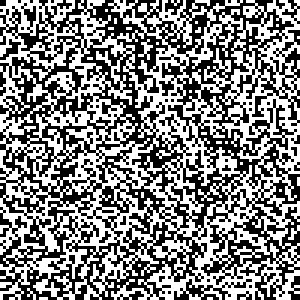# Mathematical definition

We have a lattice $$\Lambda$$ consisting of sites $$k$$. For each site, there is a moment $$\sigma_k \in \{ -1, +1 \}$$. $$\sigma = (\sigma_k)_{k\in\Lambda}$$ is called the configuration of the lattice.

The total energy of the configuration is given by the Hamiltonian $H(\sigma) = -\sum_{i\sim j} J_{ij}\, \sigma_i\, \sigma_j,$ where $$i\sim j$$ denotes neighbours, and $$J$$ is the interaction matrix.

The configuration probability is given by: $\pi_\beta(\sigma) = \frac{e^{-\beta H(\sigma)}}{Z_\beta}$ where $$\beta = (k_B T)^{-1}$$ is the inverse temperature, and $$Z_\beta$$ the normalisation constant.

For our simulation, we will use a constant interaction term $$J > 0$$. If $$\sigma_i = \sigma_j$$, the probability will be proportional to $$\exp(\beta J)$$, otherwise it would be $$\exp(\beta J)$$. Thus, adjacent spins will try to align themselves.

# Simulation

The Ising model is generally simulated using Markov Chain Monte Carlo (MCMC), with the Metropolis-Hastings algorithm.

The algorithm starts from a random configuration and runs as follows:

1. Select a site $$i$$ at random and reverse its spin: $$\sigma'_i = -\sigma_i$$
2. Compute the variation in energy (hamiltonian) $$\Delta E = H(\sigma') - H(\sigma)$$
3. If the energy is lower, accept the new configuration
4. Otherwise, draw a uniform random number $$u \in ]0,1[$$ and accept the new configuration if $$u < \min(1, e^{-\beta \Delta E})$$.

# Implementation

The simulation is in Clojure, using the Quil library (a Processing library for Clojure) to display the state of the system.

This post is “literate Clojure”, and contains core.clj. The complete project can be found on GitHub.

(ns ising-model.core
(:require [quil.core :as q]
[quil.middleware :as m]))

The application works with Quil’s functional mode, with each function taking a state and returning an updated state at each time step.

The setup function generates the initial state, with random initial spins. It also sets the frame rate. The matrix is a single vector in row-major mode. The state also holds relevant parameters for the simulation: $$\beta$$, $$J$$, and the iteration step.

(defn setup [size]
"Setup the display parameters and the initial state"
(q/frame-rate 300)
(q/color-mode :hsb)
(let [matrix (vec (repeatedly (* size size) #(- (* 2 (rand-int 2)) 1)))]
{:grid-size size
:matrix matrix
:beta 10
:intensity 10
:iteration 0}))

Given a site $$i$$, we reverse its spin to generate a new configuration state.

(defn toggle-state [state i]
"Compute the new state when we toggle a cell's value"
(let [matrix (:matrix state)]
(assoc state :matrix (assoc matrix i (* -1 (matrix i))))))

In order to decide whether to accept this new state, we compute the difference in energy introduced by reversing site $$i$$: $\Delta E = J\sigma_i \sum_{j\sim i} \sigma_j.$

The filter some? is required to eliminate sites outside of the boundaries of the lattice.

(defn get-neighbours [state idx]
"Return the values of a cell's neighbours"
[(get (:matrix state) (- idx (:grid-size state)))
(get (:matrix state) (dec idx))
(get (:matrix state) (inc idx))
(get (:matrix state) (+ (:grid-size state) idx))])

(defn delta-e [state i]
"Compute the energy difference introduced by a particular cell"
(* (:intensity state) ((:matrix state) i)
(reduce + (filter some? (get-neighbours state i)))))

We also add a function to compute directly the hamiltonian for the entire configuration state. We can use it later to log its values across iterations.

(defn hamiltonian [state]
"Compute the Hamiltonian of a configuration state"
(- (reduce + (for [i (range (count (:matrix state)))
j (filter some? (get-neighbours state i))]
(* (:intensity state) ((:matrix state) i) j)))))

Finally, we put everything together in the update-state function, which will decide whether to accept or reject the new configuration.

(defn update-state [state]
"Accept or reject a new state based on energy
difference (Metropolis-Hastings)"
(let [i (rand-int (count (:matrix state)))
new-state (toggle-state state i)
alpha (q/exp (- (* (:beta state) (delta-e state i))))]
;;(println (hamiltonian new-state))
(update (if (< (rand) alpha) new-state state)
:iteration inc)))

The last thing to do is to draw the new configuration:

(defn draw-state [state]
"Draw a configuration state as a grid"
(q/background 255)
(let [cell-size (quot (q/width) (:grid-size state))]
(doseq [[i v] (map-indexed vector (:matrix state))]
(let [x (* cell-size (rem i (:grid-size state)))
y (* cell-size  (quot i (:grid-size state)))]
(q/no-stroke)
(q/fill
(if (= 1 v) 0 255))
(q/rect x y cell-size cell-size))))
;;(when (zero? (mod (:iteration state) 50)) (q/save-frame "img/ising-######.jpg"))
)

And to reset the simulation when the user clicks anywhere on the screen:

(defn mouse-clicked [state event]
"When the mouse is clicked, reset the configuration to a random one"
(setup 100))
(q/defsketch ising-model
:title "Ising model"
:size [300 300]
:setup #(setup 100)
:update update-state
:draw draw-state
:mouse-clicked mouse-clicked
:features [:keep-on-top :no-bind-output]
:middleware [m/fun-mode])

# Conclusion

The Ising model is a really easy (and common) example use of MCMC and Metropolis-Hastings. It allows to easily and intuitively understand how the algorithm works, and to make nice visualizations!

]]>
Generating and representing L-systems https://www.lozeve.com/posts/lsystems.html 2018-01-18T00:00:00Z 2018-01-18T00:00:00Z
Posted on January 18, 2018 by Dimitri Lozeve

L-systems are a formal way to make interesting visualisations. You can use them to model a wide variety of objects: space-filling curves, fractals, biological systems, tilings, etc.

See the Github repo: https://github.com/dlozeve/lsystems

# What is an L-system?

## A few examples to get started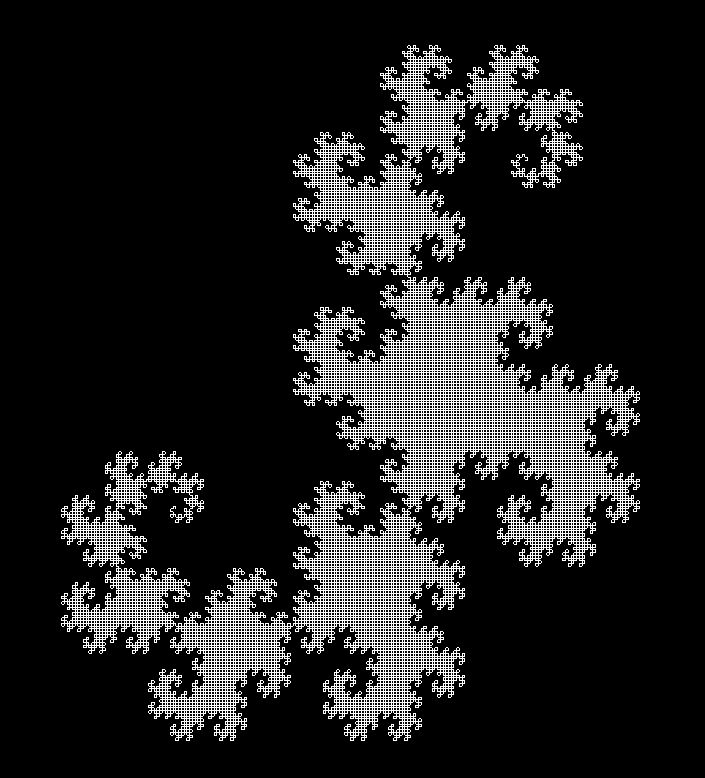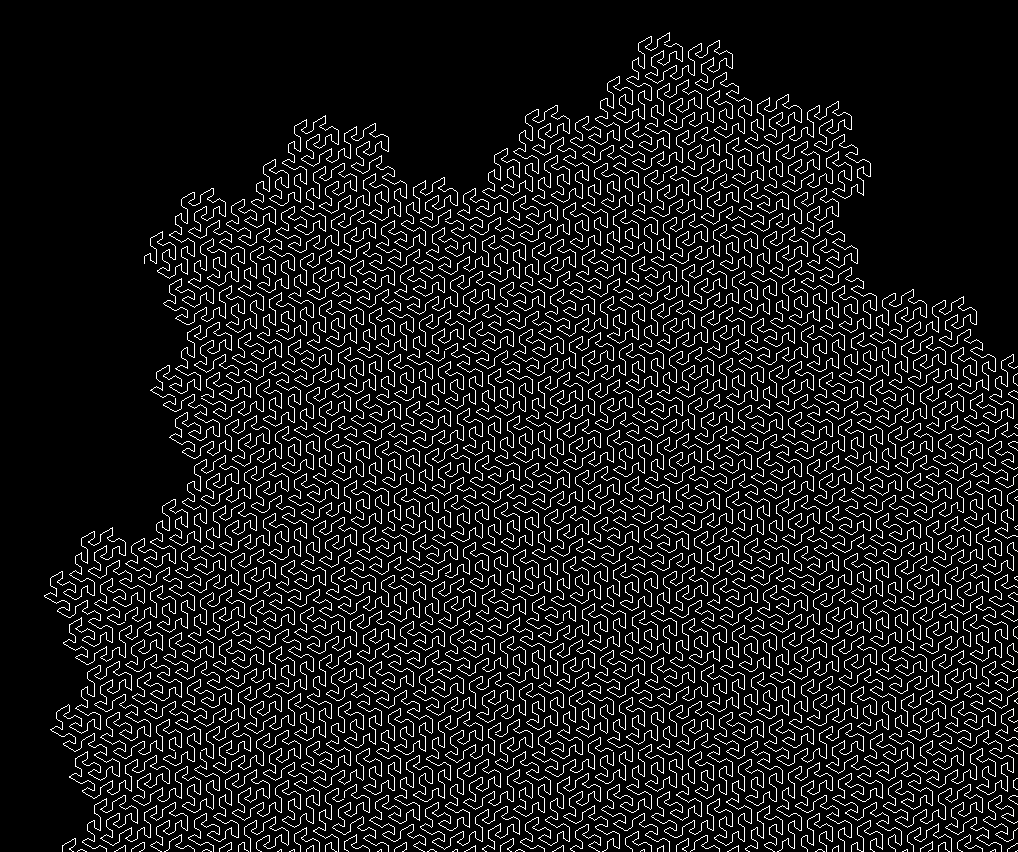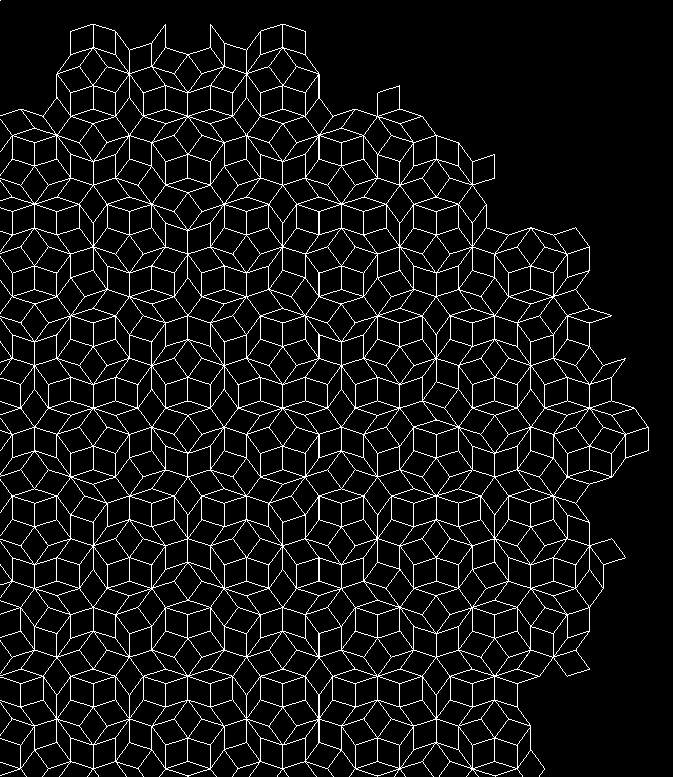## Definition

An L-system is a set of rewriting rules generating sequences of symbols. Formally, an L-system is a triplet of:

• an alphabet $$V$$ (an arbitrary set of symbols)
• an axiom $$\omega$$, which is a non-empty word of the alphabet ($$\omega \in V^+$$)
• a set of rewriting rules (or productions) $$P$$, each mapping a symbol to a word: $$P \subset V \times V^*$$. Symbols not present in $$P$$ are assumed to be mapped to themselves.

During an iteration, the algorithm takes each symbol in the current word and replaces it by the value in its rewriting rule. Not that the output of the rewriting rule can be absolutely anything in $$V^*$$, including the empty word! (So yes, you can generate symbols just to delete them afterwards.)

At this point, an L-system is nothing more than a way to generate very long strings of characters. In order to get something useful out of this, we have to give them meaning.

## Drawing instructions and representation

Our objective is to draw the output of the L-system in order to visually inspect the output. The most common way is to interpret the output as a sequence of instruction for a LOGO-like drawing turtle. For instance, a simple alphabet consisting only in the symbols $$F$$, $$+$$, and $$-$$ could represent the instructions “move forward”, “turn right by 90°”, and “turn left by 90°” respectively.

Thus, we add new components to our definition of L-systems:

• a set of instructions, $$I$$. These are limited by the capabilities of our imagined turtle, so we can assume that they are the same for every L-system we will consider:
• Forward makes the turtle draw a straight segment.
• TurnLeft and TurnRight makes the turtle turn on itself by a given angle.
• Push and Pop allow the turtle to store and retrieve its position on a stack. This will allow for branching in the turtle’s path.
• Stay, which orders the turtle to do nothing.
• a distance $$d \in \mathbb{R_+}$$, i.e. how long should each forward segment should be.
• an angle $$\theta$$ used for rotation.
• a set of representation rules $$R \subset V \times I$$. As before, they will match a symbol to an instruction. Symbols not matched by any rule will be associated to Stay.

Finally, our complete L-system, representable by a turtle with capabilities $$I$$, can be defined as $L = (V, \omega, P, d, \theta, R).$

One could argue that the representation is not part of the L-system, and that the same L-system could be represented differently by changing the representation rules. However, in our setting, we won’t observe the L-system other than by displaying it, so we might as well consider that two systems differing only by their representation rules are different systems altogether.

# Implementation details

## The LSystem data type

The mathematical definition above translate almost immediately in a Haskell data type:

-- | L-system data type
data LSystem a = LSystem
{ name :: String
, alphabet :: [a] -- ^ variables and constants used by the system
, axiom :: [a] -- ^ initial state of the system
, rules :: [(a, [a])] -- ^ production rules defining how each
-- variable can be replaced by a sequence of
-- variables and constants
, angle :: Float -- ^ angle used for the representation
, distance :: Float -- ^ distance of each segment in the representation
, representation :: [(a, Instruction)] -- ^ representation rules
-- defining how each variable
-- and constant should be
-- represented
} deriving (Eq, Show, Generic)

Here, a is the type of the literal in the alphabet. For all practical purposes, it will almost always be Char.

Instruction is just a sum type over all possible instructions listed above.

## Iterating and representing

From here, generating L-systems and iterating is straightforward. We iterate recursively by looking up each symbol in rules and replacing it by its expansion. We then transform the result to a list of Instruction.

## Drawing

The only remaining thing is to implement the virtual turtle which will actually execute the instructions. It goes through the list of instructions, building a sequence of points and maintaining an internal state (position, angle, stack). The stack is used when Push and Pop operations are met. In this case, the turtle builds a separate line starting from its current position.

The final output is a set of lines, each being a simple sequence of points. All relevant data types are provided by the Gloss library, along with the function that can display the resulting Picture.

# Common file format for L-systems

In order to define new L-systems quickly and easily, it is necessary to encode them in some form. We chose to represent them as JSON values.

Here is an example for the Gosper curve:

{
"name": "gosper",
"alphabet": "AB+-",
"axiom": "A",
"rules": [
["A", "A-B--B+A++AA+B-"],
["B", "+A-BB--B-A++A+B"]
],
"angle": 60.0,
"distance": 10.0,
"representation": [
["A", "Forward"],
["B", "Forward"],
["+", "TurnRight"],
["-", "TurnLeft"]
]
}

Using this format, it is easy to define new L-systems (along with how they should be represented). This is translated nearly automatically to the LSystem data type using Aeson.

# Variations on L-systems

We can widen the possibilities of L-systems in various ways. L-systems are in effect deterministic context-free grammars.

By allowing multiple rewriting rules for each symbol with probabilities, we can extend the model to probabilistic context-free grammars.

We can also have replacement rules not for a single symbol, but for a subsequence of them, thus effectively taking into account their neighbours (context-sensitive grammars). This seems very close to 1D cellular automata.

Finally, L-systems could also have a 3D representation (for instance space-filling curves in 3 dimensions).

# Usage notes

1. Clone the repository: git clone [[https://github.com/dlozeve/lsystems]]
2. Build: stack build
3. Execute stack exec lsystems-exe -- examples/penroseP3.json to see the list of options
4. (Optional) Run tests and build documentation: stack test --haddock

Usage: stack exec lsystems-exe -- --help

lsystems -- Generate L-systems

Usage: lsystems-exe FILENAME [-n|--iterations N] [-c|--color R,G,B]
[-w|--white-background]
Generate and draw an L-system

Available options:
FILENAME                 JSON file specifying an L-system
-n,--iterations N        Number of iterations (default: 5)
-c,--color R,G,B         Foreground color RGBA
(0-255) (default: RGBA 1.0 1.0 1.0 1.0)
-w,--white-background    Use a white background
-h,--help                Show this help text


Apart from the selection of the input JSON file, you can adjust the number of iterations and the colors.

stack exec lsystems-exe -- examples/levyC.json -n 12 -c 0,255,255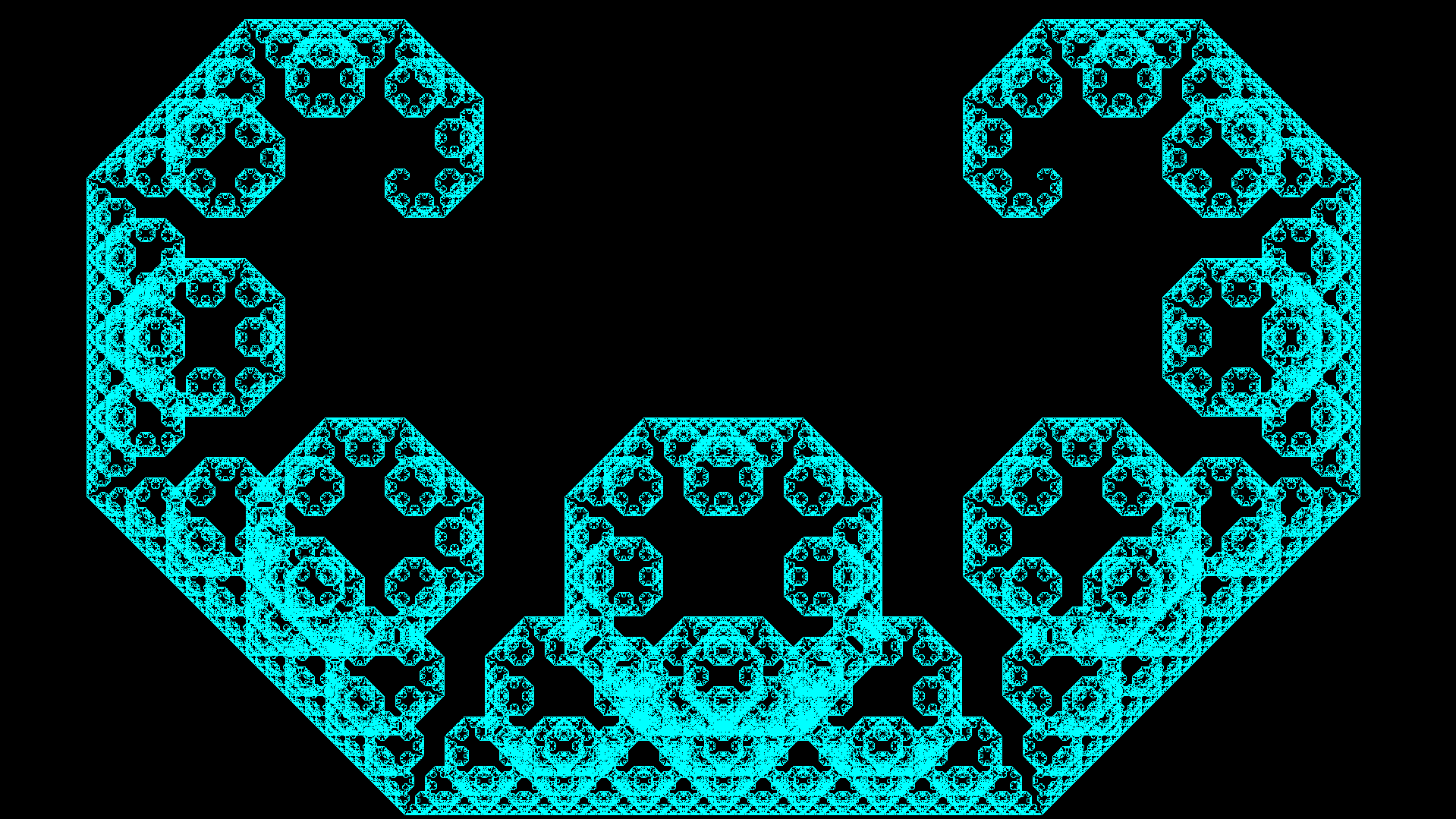# References

1. Prusinkiewicz, Przemyslaw; Lindenmayer, Aristid (1990). The Algorithmic Beauty of Plants. Springer-Verlag. ISBN 978-0-387-97297-8. http://algorithmicbotany.org/papers/#abop
2. Weisstein, Eric W. “Lindenmayer System.” From MathWorld–A Wolfram Web Resource. http://mathworld.wolfram.com/LindenmayerSystem.html
3. Corte, Leo. “L-systems and Penrose P3 in Inkscape.” The Brick in the Sky. https://thebrickinthesky.wordpress.com/2013/03/17/l-systems-and-penrose-p3-in-inkscape/
]]>